mscroggs.co.uk
mscroggs.co.uksubscribe

# Blog

2020-12-03
In November, I spent some time designing this year's Chalkdust puzzle Christmas card.
The card looks boring at first glance, but contains 9 puzzles. By splitting the answers into two digit numbers, then colouring the regions labelled with each number (eg if an answer to a question in the red section is 201304, colour the regions labelled 20, 13 and 4 red), you will reveal a Christmas themed picture.
If you want to try the card yourself, you can download this pdf. Alternatively, you can find the puzzles below and type the answers in the boxes. The answers will be automatically be split into two digit numbers, and the regions will be coloured...

### Similar postsChristmas card 2019Christmas card 2018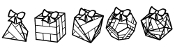Christmas card 2017Christmas card 2016

Comments in green were written by me. Comments in blue were not written by me.
@JDev: lots of the card will still be brown once you're done, but you should see a nice picture. Perhaps one of your answers is wrong, making a mess of the picture?
Matthew
I finished all of the puzzles but the picture is far from colored in. Am I missing something?

These puzzles have been a blast!
JDev
@Tara: I initially made the same mistake. Maybe you didn't take into account that 6 is not one of the available digits in question 1?
Sean
@Tara: Yes, looks like you may have got an incorrect answer for one of the black puzzles
Matthew

Allowed HTML tags: <br> <a> <small> <b> <i> <s> <sup> <sub> <u> <spoiler> <ul> <ol> <li>
To prove you are not a spam bot, please type "o" then "d" then "d" in the box below (case sensitive):
2019-12-08
Just like last year, the year before and the year before, TD and I spent some time in November this year designing a Chalkdust puzzle Christmas card.
The card looks boring at first glance, but contains 9 puzzles. By splitting the answers into two digit numbers, then drawing lines labelled with each number (eg if an answer is 201304, draw the lines labelled 20, 13 and 4), you will reveal a Christmas themed picture. Colouring the regions of the card containing circles red, the regions containing squares green, and the regions containing stars white or yellow will make this picture even nicer.
If you want to try the card yourself, you can download this pdf. Alternatively, you can find the puzzles below and type the answers in the boxes. The answers will be automatically be split into two digit numbers, the lines will be drawn, and the regions will be coloured...

### Similar posts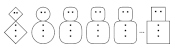Christmas card 2020Christmas card 2018Christmas card 2017Christmas card 2016

Comments in green were written by me. Comments in blue were not written by me.
Thanks for the feedback. (I now understand the need for redaction). My son sent me your link as a Xmas present. I must think of an appropriate retaliation. (What is a PDF?)Think I've fixed 1,6 and 9....8 eludes me, for the moment.
Rob
@Rob: It looks to me like you've made mistakes in questions 1, 6, 8, and 9. The hints from the back of the pdf might help:

1. How many numbers between 1 and 10,000 have 1 as their final digit? How many have 1 as their penultimate digit?

6. How many ways can you write 1? 2? 3? 4? 5? What's the pattern?

8. How many zeros does 10! end in? How many zeros does 20! end in? How many zeros does 30! end in?

9. Carol’s sum is odd. What does this tell you about the 5- and 6-digit numbers?
Matthew
I'm 71, with one good eye left. What am I missing?
1. 400001
2. 1849
3. 2002
4. 130405
5. 120306
6. 53?
7. 171175
8. 59?
9. 313525
Rob

Allowed HTML tags: <br> <a> <small> <b> <i> <s> <sup> <sub> <u> <spoiler> <ul> <ol> <li>
To prove you are not a spam bot, please type "sexa" backwards in the box below (case sensitive):
2019-09-01
This week, I've been in Cambridge for Talking Maths in Public (TMiP). TMiP is a conference for anyone involved in—or interested in getting involved in—any sort of maths outreach, enrichment, or public engagement activity. It was really good, and I highly recommend coming to TMiP 2021.
The Saturday morning at TMiP was filled with a choice of activities, including a treasure punt (a treasure hunt on a punt) written by me. This post contains the puzzle from the treasure punt for anyone who was there and would like to revisit it, or anyone who wasn't there and would like to give it a try. In case you're not current in Cambridge on a punt, the clues that you were meant to spot during the punt are given behing spoiler tags (hover/click to reveal).

### Instructions

Each boat was given a copy of the instructions, and a box that was locked using a combination lock.
If you want to make your own treasure punt or similar activity, you can find the LaTeX code used to create the instructions and the Python code I used to check that the puzzle has a unique solution on GitHub. It's licensed with a CC BY 4.0 licence, so you can resuse an edit it in any way you like, as long as you attribute the bits I made that you keep.

### The puzzle

Four mathematicians—Ben, Katie, Kevin, and Sam—each have one of the four clues needed to unlock a great treasure. On a sunny/cloudy/rainy/snowy (delete as appropriate) day, they meet up in Cambridge to go punting, share their clues, work out the code for the lock, and share out the treasure. One or more of the mathematicians, however, has decided to lie about their clue so they can steal all the treasure for themselves. At least one mathematician is telling the truth. (If the mathematicians say multiple sentences about their clue, then they are either all true or all false.)
They meet at Cambridge Chauffeur Punts, and head North under Silver Street Bridge. Ben points out a plaque on the bridge with two years written on it:
"My clue," he says, "tells me that the sum of the digits of the code is equal to the sum of the digits of the earlier year on that plaque (the year is 1702). My clue also tells me that at least one of the digits of the code is 7."
The mathematicians next punt under the Mathematical Bridge, gasping in awe at its tangential trusses, then punt along the river under King's College Bridge and past King's College. Katie points to a sign on the King's College lawn near the river:
"See that sign whose initials are PNM?" says Katie. "My clue states that first digit of the code is equal to the number of vowels on that sign (The sign says "Private: No Mooring"). My clue also tells me that at least one of the digits of the code is 1."
They then reach Clare Bridge. Kevin points out the spheres on Clare Bridge:
"My clue," he says, "states that the total number of spheres on both sides of this bridge is a factor of the code (there are 14 spheres). My clue also tells me that at least one of the digits of the code is 2." (Kevin has not noticed that one of the spheres had a wedge missing, so counts that as a whole sphere.)
They continue past Clare College. Just before they reach Garret Hostel Bridge, Sam points out the Jerwood Library and a sign showing the year it was built (it was built in 1998):
"My clue," she says, "says that the largest prime factor of that year appears in the code (in the same way that you might say the number 18 appears in 1018 or 2189). My clue also says that the smallest prime factor of that year appears in the code. My clue also told me that at least one of the digits of the code is 0."
They then punt under Garret Hostel Bridge, turn around between it and Trinity College Bridge, and head back towards Cambridge Chauffeur Punts. Zut alors, the lies confuse them and they can't unlock the treasure. Can you work out who is lying and claim the treasure for yourself?

### The solution

The solution to the treasure punt is given below. Once you're ready to see it, click "Show solution".

### Similar postsChristmas card 2020Christmas card 2019Christmas card 2018Christmas card 2017

Comments in green were written by me. Comments in blue were not written by me.

Allowed HTML tags: <br> <a> <small> <b> <i> <s> <sup> <sub> <u> <spoiler> <ul> <ol> <li>
To prove you are not a spam bot, please type "orez" backwards in the box below (case sensitive):
2019-04-09
In the latest issue of Chalkdust, I wrote an article with Edmund Harriss about the Harriss spiral that appears on the cover of the magazine. To draw a Harriss spiral, start with a rectangle whose side lengths are in the plastic ratio; that is the ratio $$1:\rho$$ where $$\rho$$ is the real solution of the equation $$x^3=x+1$$, approximately 1.3247179.
This rectangle can be split into a square and two rectangles similar to the original rectangle. These smaller rectangles can then be split up in the same manner.
Drawing two curves in each square gives the Harriss spiral.
This spiral was inspired by the golden spiral, which is drawn in a rectangle whose side lengths are in the golden ratio of $$1:\phi$$, where $$\phi$$ is the positive solution of the equation $$x^2=x+1$$ (approximately 1.6180339). This rectangle can be split into a square and one similar rectangle. Drawing one arc in each square gives a golden spiral.
The golden and Harriss spirals are both drawn in rectangles that can be split into a square and one or two similar rectangles.
Continuing the pattern of these arrangements suggests the following rectangle, split into a square and three similar rectangles:
Let the side of the square be 1 unit, and let each rectangle have sides in the ratio $$1:x$$. We can then calculate that the lengths of the sides of each rectangle are as shown in the following diagram.
The side lengths of the large rectangle are $$\frac{1}{x^3}+\frac{1}{x^2}+\frac2x+1$$ and $$\frac1{x^2}+\frac1x+1$$. We want these to also be in the ratio $$1:x$$. Therefore the following equation must hold:
$$\frac{1}{x^3}+\frac{1}{x^2}+\frac2x+1=x\left(\frac1{x^2}+\frac1x+1\right)$$
Rearranging this gives:
$$x^4-x^2-x-1=0$$ $$(x+1)(x^3-x^2-1)=0$$
This has one positive real solution:
$$x=\frac13\left( 1 +\sqrt{\tfrac12(29-3\sqrt{93})} +\sqrt{\tfrac12(29+3\sqrt{93})} \right).$$
This is equal to 1.4655712... Drawing three arcs in each square allows us to make a spiral from a rectangle with sides in this ratio: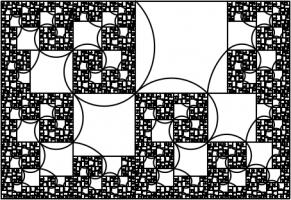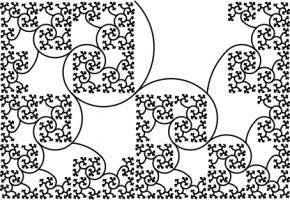A spiral which may or may not have a name yet.

### Continuing the pattern

The side lengths of the largest rectangle are $$1+\frac2x+\frac3{x^2}+\frac1{x^3}+\frac1{x^4}$$ and $$1+\frac2x+\frac1{x^2}+\frac1{x^3}$$. Looking for the largest rectangle to also be in the ratio $$1:x$$ leads to the equation:
$$1+\frac2x+\frac3{x^2}+\frac1{x^3}+\frac1{x^4} = x\left(1+\frac2x+\frac1{x^2}+\frac1{x^3}\right)$$ $$x^5+x^4-x^3-2x^2-x-1 = 0$$
This has one real solution, 1.3910491... Although for this rectangle, it's not obvious which arcs to draw to make a spiral (or maybe not possible to do it at all). But at least you get a pretty fractal: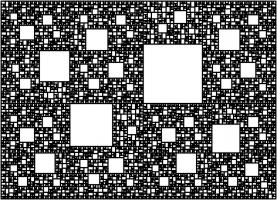### Continuing the pattern

We could, of course, continue the pattern by repeatedly adding more rectangles. If we do this, we get the following polynomials and solutions:
 Number of rectangles Polynomial Solution 1 $$x^2 - x - 1=0$$ 1.618033988749895 2 $$x^3 - x - 1=0$$ 1.324717957244746 3 $$x^4 - x^2 - x - 1=0$$ 1.465571231876768 4 $$x^5 + x^4 - x^3 - 2x^2 - x - 1=0$$ 1.391049107172349 5 $$x^6 + x^5 - 2x^3 - 3x^2 - x - 1=0$$ 1.426608021669601 6 $$x^7 + 2x^6 - 2x^4 - 3x^3 - 4x^2 - x - 1=0$$ 1.4082770325090774 7 $$x^8 + 2x^7 + 2x^6 - 2x^5 - 5x^4 - 4x^3 - 5x^2 - x - 1=0$$ 1.4172584399350432 8 $$x^9 + 3x^8 + 2x^7 - 5x^5 - 9x^4 - 5x^3 - 6x^2 - x - 1=0$$ 1.412713760332943 9 $$x^{10} + 3x^9 + 5x^8 - 5x^6 - 9x^5 - 14x^4 - 6x^3 - 7x^2 - x - 1=0$$ 1.414969877544769
The numbers in this table appear to be heading towards around 1.414, or $$\sqrt2$$. This shouldn't come as too much of a surprise because $$1:\sqrt2$$ is the ratio of the sides of A$$n$$ paper (for $$n=0,1,2,...$$). A0 paper can be split up like this:
This is a way of splitting up a $$1:\sqrt{2}$$ rectangle into an infinite number of similar rectangles, arranged following the pattern, so it makes sense that the ratios converge to this.

### Other patterns

In this post, we've only looked at splitting up rectangles into squares and similar rectangles following a particular pattern. Thinking about other arrangements leads to the following question:
Given two real numbers $$a$$ and $$b$$, when is it possible to split an $$a:b$$ rectangle into squares and $$a:b$$ rectangles?
If I get anywhere with this question, I'll post it here. Feel free to post your ideas in the comments below.

### Similar posts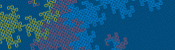Dragon curves IIChristmas card 2020A surprising fact about quadrilateralsChristmas card 2019

Comments in green were written by me. Comments in blue were not written by me.
@g0mrb: CORRECTION: There seems to be no way to correct the glaring error in that comment. A senior moment enabled me to reverse the nomenclature for paper sizes. Please read the suffixes as (n+1), (n+2), etc.
(anonymous)
I shall remain happy in the knowledge that you have shown graphically how an A(n) sheet, which is 2 x A(n-1) rectangles, is also equal to the infinite series : A(n-1) + A(n-2) + A(n-3) + A(n-4) + ... Thank-you, and best wishes for your search for the answer to your question.
g0mrb

Allowed HTML tags: <br> <a> <small> <b> <i> <s> <sup> <sub> <u> <spoiler> <ul> <ol> <li>
To prove you are not a spam bot, please type "naidem" backwards in the box below (case sensitive):
2018-12-08
Just like last year and the year before, TD and I spent some time in November this year designing a Chalkdust puzzle Christmas card.
The card looks boring at first glance, but contains 10 puzzles. By splitting the answers into pairs of digits, then drawing lines between the dots on the cover for each pair of digits (eg if an answer is 201304, draw a line from dot 20 to dot 13 and another line from dot 13 to dot 4), you will reveal a Christmas themed picture. Colouring the region of the card labelled R red or orange will make this picture even nicer.
If you want to try the card yourself, you can download this pdf. Alternatively, you can find the puzzles below and type the answers in the boxes. The answers will be automatically be split into pairs of digits, lines will be drawn between the pairs, and the red region will be coloured...
If you enjoy these puzzles, then you'll almost certainly enjoy this year's puzzle Advent calendar.

### Similar postsChristmas card 2020Christmas card 2019Christmas card 2017Christmas card 2016

Comments in green were written by me. Comments in blue were not written by me.
Someone told me I would like this puzzle and they were right!
Blaine
@Carmel: It's not meant to check your answers. It only shows up red if the number you enter cannot be split into valid pairs (eg the number has an odd number of digits or one of the pairs of digits is greater than 20).
Matthew
The script for checking the answers doesn't work properly
Carmel
Great puzzle problems! Hint on #9: try starting with an analogous problem using smaller numbers (e.g. 3a + 10b). This helped me to see what I had to do more generally.
Noah